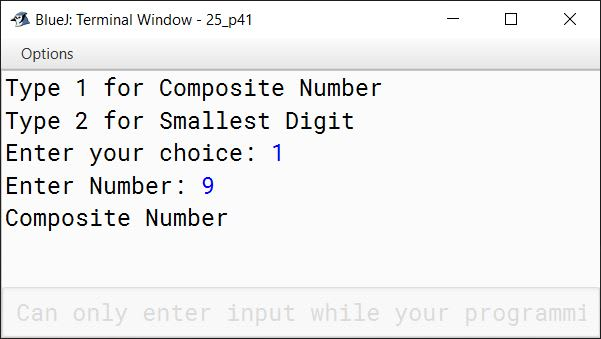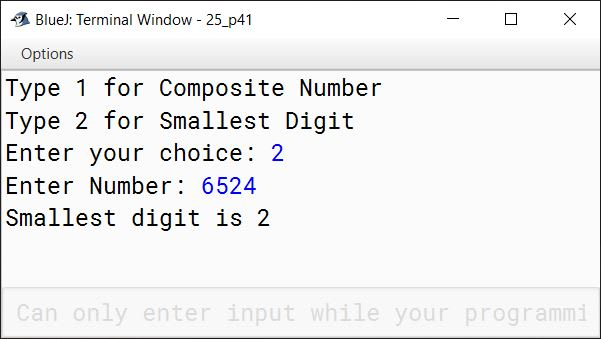# Conditional Constructs in Java

## Multiple Choice Questions

#### Question 1

Which operator cannot be used with if-else statement?

1. <=
2. ||
3. &&
4. ? : ✓

#### Question 2

Predict the output of the following code snippet:

int a = 1;
int b = 2;
if (a == b)
System.out.println ("Both values are equal");
else
System.out.println ("Values are not equal");
1. Both values are equal
2. Incorrect use of the == operator
3. Values are not equal ✓
4. No output

#### Question 3

Consider the following code snippet:

if ( c > d)
x = c;
else
x = d;

Choose the correct option if the code mentioned above is rewritten using the ternary operator:

1. x = (c >d) ? c : d; ✓
2. x = (c >d) ? d : c;
3. x = (c >d) ? c : c;
4. x = (c >d) ? d : d;

#### Question 4

if ((a > b) && (a > c)), then which of the following statements is true?

1. a is the largest number. ✓
2. b is the largest number.
3. c is the largest number.
4. b is the smallest number.

#### Question 5

Consider the following code snippet:

int val = 2;
switch (val)
{
case 1: System.out.println("Case 1");
break;
case 2: System.out.println("Case 2");
break;
default: System.out.println("No match found");
break;
}

Which of the following statements is correct?

1. case 1 will be executed.
2. case 2 will be executed. ✓
3. default will be executed.
4. both case 1 and 2 will be executed.

#### Question 6

A sequence of statements enclosed between a pair of curly brackets is called

1. a compound statement ✓
2. an empty statement
3. a null statement
4. a void statement

#### Question 7

Which clause is optional in the switch statement?

1. default ✓
2. case
3. switch
4. None of these

#### Question 8

Which of the following statements involves a fall-through?

1. if-else
2. for loop
4. switch ✓

#### Question 9

Which of the following causes a fall-through in the switch statement?

1. the omission of fall
2. the omission of continue
3. the omission of break ✓
4. the omission of loop

#### Question 10

Which of the following is mandatory in the switch statement?

1. break
2. continue
3. case ✓
4. default

## Assignment Questions

#### Question 1

Explain the significance of break statement in the switch statement.

The break statement is used inside the switch statement to terminate a statement block. It brings the program control out of the switch statement.

#### Question 2

Explain the significance of the default label in the switch statement.

When none of the case values are equal to the expression of switch statement then default case is executed. In the example below, value of number is 4 so case 0, case 1 and case 2 are not equal to number. Hence println statement of default case will get executed printing "Value of number is greater than two" to the console.

int number = 4;
switch(number) {
case 0:
System.out.println("Value of number is zero");
break;
case 1:
System.out.println("Value of number is one");
break;
case 2:
System.out.println("Value of number is two");
break;
default:
System.out.println("Value of number is greater than two");
break;
}
##### Output
Value of number is greater than two


#### Question 3

Format the following if statements with indentation:

i. if (x == y) if (x == z) x = 1; else y = 1; else z = 1;

if (x == y)
if (x == z)
x = 1;
else
y = 1;
else
z = 1;

ii. if (x == y) {if (y == z) x = 1; y = 2; } else z = 1;

if (x == y)
{
if (y == z)
x = 1;
y = 2;
}
else
z = 1;

iii. if (num1 != num2) {
if (num2 >= num3) x = 1; y = 2; }
else {x = 1; if (num1 == num2) z = 3;}

if (num1 != num2)
{
if (num2 >= num3)
x = 1;
y = 2;
}
else
{
x = 1;
if (num1 == num2)
z = 3;
}

#### Question 4

Rewrite the following if statement, using the switch statement:

if (choice == 0)
System.out.println("You selected Blue");
else if (choice == 1)
System.out.println("You selected Cyan");
else if (choice == 2)
System.out.println("You selected Red");
else if (choice == 3)
System.out.println("You selected Magenta");
else if (choice == 4)
System.out.println("You selected Green");
else if (choice == 5)
System.out.println("You selected Yellow");
else
System.out.println("Invalid choice");

switch (choice) {
case 1:
System.out.println("You selected Blue");
break;

case 2:
System.out.println("You selected Cyan");
break;

case 3:
System.out.println("You selected Red");
break;

case 4:
System.out.println("You selected Magenta");
break;

case 5:
System.out.println("You selected Green");
break;

case 6:
System.out.println("You selected Yellow");
break;

default:
System.out.println("Invalid choice");
}

#### Question 5

Write the following switch statement by using nested if statements:

switch (choice)
{
case 0:
case 1:
x = 11;
y = 22;
break;
case 2:
x = 33;
y = 44;
break;
default:
y = 55;
break;
}

if (choice == 0 || choice == 1)
{
x = 11;
y = 22;
}
else
{
if (choice == 2)
{
x = 33;
y = 44;
}
else
{
y = 55;
}
}

#### Question 6

Write an if statement to find the smallest of the three given integers using the min() method of the Math class.

if (a < Math.min(b, c))
System.out.println(a);
else
System.out.println(Math.min(b, c));

#### Question 7

Using the switch statement in Java, write a program to display the colour of the spectrum (VIBGYOR) according to the user's choice.

import java.util.Scanner;

public class KboatSpectrum
{
public static void main(String args[]) {
Scanner in = new Scanner(System.in);
System.out.println("VIBGYOR Spectrum");
char choice = in.next().charAt(0);
switch (choice) {
case 'V':
System.out.println("Violet");
break;

case 'I':
System.out.println("Indigo");
break;

case 'B':
System.out.println("Blue");
break;

case 'G':
System.out.println("Green");
break;

case 'Y':
System.out.println("Yellow");
break;

case 'O':
System.out.println("Orange");
break;

case 'R':
System.out.println("Red");
break;

default:
System.out.println("Incorrect choice");
}
}
}
##### Output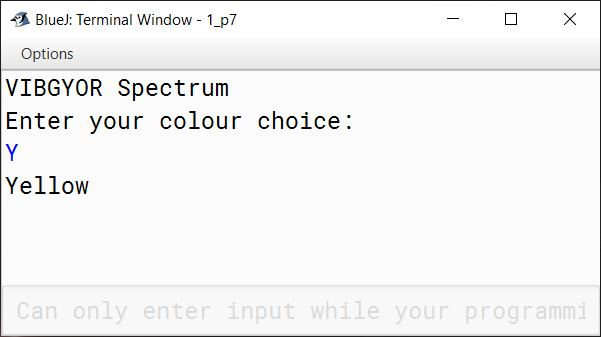#### Question 8

Employees at Arkenstone Consulting earn the basic hourly wage of Rs.500. In addition to this, they also receive a commission on the sales they generate while tending the counter. The commission given to them is calculated according to the following table:

Total SalesCommmision Rate
Rs. 100 to less than Rs. 10001%
Rs. 1000 to less than Rs. 100002%
Rs. 10000 to less than Rs. 250003%
Rs. 25000 and above3.5%

Write a program in Java that inputs the number of hours worked and the total sales. Compute the wages of the employees.

import java.util.Scanner;

public class KboatArkenstoneConsulting
{
public static void main(String args[]) {
Scanner in = new Scanner(System.in);
System.out.print("Enter number of hours: ");
int hrs = in.nextInt();
System.out.print("Enter total sales: ");
int sales = in.nextInt();
double wage = hrs * 500;
double c = 0;
if (sales < 100)
c = 0;
else if (sales < 1000)
c = 1;
else if (sales < 10000)
c = 2;
else if (sales < 25000)
c = 3;
else
c = 3.5;

double comm = c * sales / 100.0;
wage += comm;

System.out.println("Wage = " + wage);
}
}
##### Output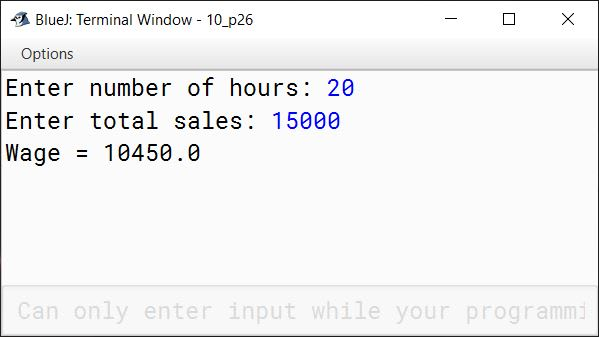#### Question 9

Write a program in Java to compute the perimeter and area of a triangle, with its three sides given as a, b, and c using the following formulas:

$\text{Perimeter} = a + b + c$

$\text{Area} = \sqrt{s(s - a)(s - b)(s - c)}$

$\text{Where} \space s = \dfrac{a+b+c}{2}$

import java.util.Scanner;

public class KboatTriangle
{
public static void main(String args[]) {
Scanner in = new Scanner(System.in);
System.out.print("Enter first side: ");
double a = in.nextDouble();
System.out.print("Enter second side: ");
double b = in.nextDouble();
System.out.print("Enter third side: ");
double c = in.nextDouble();

double perimeter = a + b + c;
double s = perimeter / 2;
double area = Math.sqrt(s * (s - a) * (s - b) * (s - c));

System.out.println("Perimeter = " + perimeter);
System.out.println("Area = " + area);
}
}
##### Output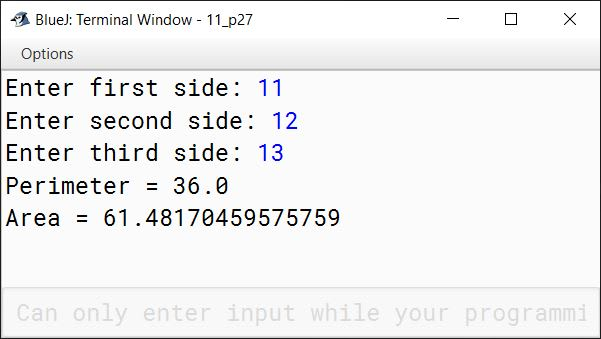#### Question 10

Mayur Transport Company charges for parcels as per the following tariff:

WeightCharges
Upto 10 Kg.Rs. 30 per Kg.
For the next 20 Kg.Rs. 20 per Kg.
Above 30 Kg.Rs. 15 per Kg.

Write a program in Java to calculate the charge for a parcel, taking the weight of the parcel as an input.

import java.util.Scanner;

public class KboatMayurTpt
{
public static void main(String args[]) {
Scanner in = new Scanner(System.in);
System.out.print("Enter parcel weight: ");
double wt = in.nextDouble();
double amt = 0;

if (wt <= 10)
amt = 30 * wt;
else if (wt <= 30)
amt = 300 + ((wt - 10) * 20);
else
amt = 300 + 400 + ((wt - 30) * 15);

System.out.println("Parcel Charge = " + amt);
}
}
##### Output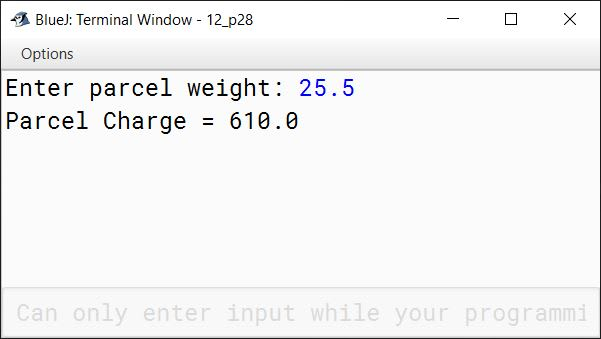#### Question 11

The electricity board charges the bill according to the number of units consumed and the rate as given below:

Units ConsumedRate Per Unit
First 100 units80 Paisa per unit
Next 200 unitsRs. 1 per unit
Above 300 unitsRs. 2.50 per unit

Write a program in Java to accept the total units consumed by a customer and calculate the bill. Assume that a meter rent of Rs. 500 is charged from the customer.

import java.util.Scanner;

public class KboatElectricBill
{
public static void main(String args[]) {
Scanner in = new Scanner(System.in);
System.out.print("Enter Units Consumed: ");
int units = in.nextInt();
double amt = 0.0;
if (units <= 100)
amt = units * 0.8;
else if (units <= 300)
amt = (100 * 0.8) + ((units - 100) * 1);
else
amt = (100 * 0.8) + (200 * 1.0) + ((units - 300) * 2.5);

amt += 500;

System.out.println("Units Consumed: " + units);
System.out.println("Bill Amount: " + amt);
}
}
##### Output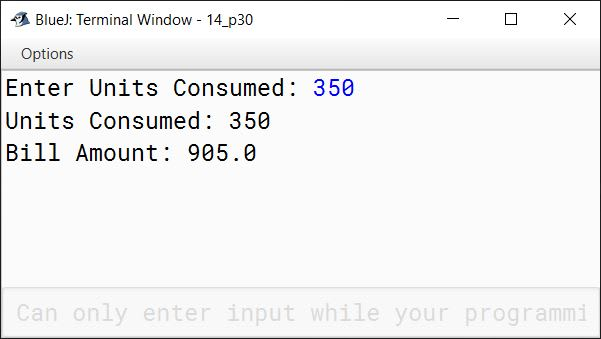#### Question 12

Write a menu driven program to accept a number from the user and check whether it is a Buzz number or an Automorphic number.

i. Automorphic number is a number, whose square's last digit(s) are equal to that number. For example, 25 is an automorphic number, as its square is 625 and 25 is present as the last two digits.
ii. Buzz number is a number, that ends with 7 or is divisible by 7.

import java.util.Scanner;

public class KboatBuzzAutomorphic
{
public static void main(String args[]) {
Scanner in = new Scanner(System.in);
System.out.println("1. Buzz number");
System.out.println("2. Automorphic number");
int choice = in.nextInt();
System.out.print("Enter number: ");
int num = in.nextInt();

switch (choice) {
case 1:
if (num % 10 == 7 || num % 7 == 0)
System.out.println(num + " is a Buzz Number");
else
System.out.println(num + " is not a Buzz Number");
break;

case 2:
int sq = num * num;
int d = 0;
int t = num;

/*
* Count the number of
* digits in num
*/
while(t > 0) {
d++;
t /= 10;
}

/*
* Extract the last d digits
* from square of num
*/
int ld = (int)(sq % Math.pow(10, d));

if (ld == num)
System.out.println(num + " is automorphic");
else
System.out.println(num + " is not automorphic");
break;

default:
System.out.println("Incorrect Choice");
break;
}
}
}
##### Output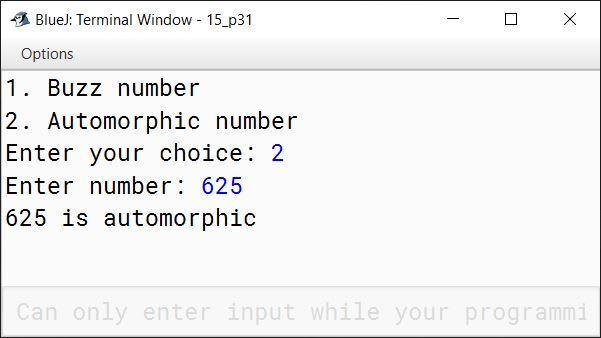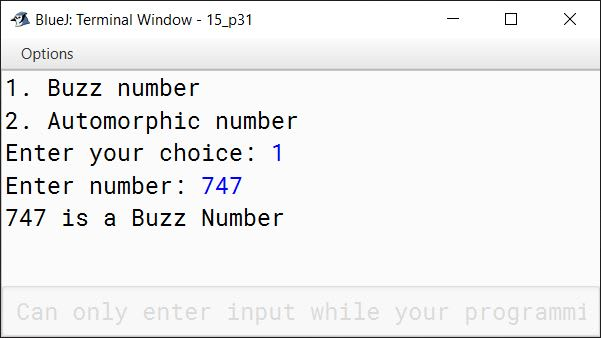#### Question 13

Write a program in Java to accept three numbers and check whether they are Pythagorean Triplet or not. The program must display the message accordingly. [Hint: h2=p2+b2]

import java.util.Scanner;

public class KboatPythagoreanTriplet
{
public static void main(String args[]) {
Scanner in = new Scanner(System.in);
System.out.print("Enter 1st number: ");
int a = in.nextInt();
System.out.print("Enter 2nd number: ");
int b = in.nextInt();
System.out.print("Enter 3rd number: ");
int c = in.nextInt();

if (a * a + b * b == c * c ||
a * a + c * c == b * b ||
b * b + c * c == a * a)
System.out.println("Pythagorean Triplets");
else
System.out.println("Not Pythagorean Triplets");
}
}
##### Output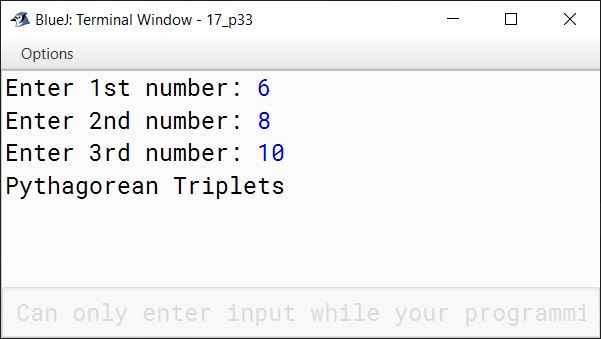#### Question 14

A cloth showroom has announced the following festival discounts on the purchase of items based on the total cost of the items purchased:

Total CostDiscount Rate
Less than Rs. 20005%
Rs. 2000 to less than Rs. 500025%
Rs. 5000 to less than Rs. 10,00035%
Rs. 10,000 and above50%

Write a program to input the total cost and to compute and display the amount to be paid by the customer availing the the discount.

import java.util.Scanner;

public class KboatClothDiscount
{
public static void main(String args[]) {
Scanner in = new Scanner(System.in);
System.out.print("Enter total cost: ");
double cost = in.nextDouble();
double amt;

if (cost < 2000) {
amt = cost - (cost * 5 / 100.0);
}
else if (cost < 5000) {
amt = cost - (cost * 25 / 100.0);
}
else if (cost < 10000) {
amt = cost - (cost * 35 / 100.0);
}
else {
amt = cost - (cost * 50 / 100.0);
}

System.out.println("Amount to be paid: " + amt);

}
}
##### Output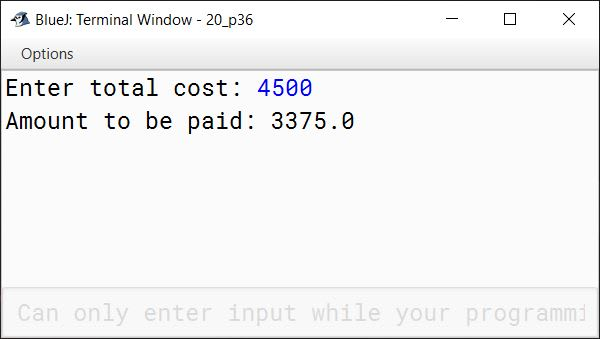#### Question 15

A box of cookies can hold 24 cookies, and a container can hold 75 boxes of cookies. Write a program that prompts the user to enter the total number of cookies, the number of cookies in each box, and the number of cookies boxes in a container. The program then outputs the number of boxes and the number of containers required to ship the cookies.

import java.util.Scanner;

{
public static void main(String args[]) {
Scanner in = new Scanner(System.in);
System.out.print("Enter total no. of cookies: ");
System.out.print("Enter no. of cookies per box: ");
System.out.print("Enter no. of boxes per container: ");
int boxPerContainer = in.nextInt();

numBox++;

int numContainer = numBox / boxPerContainer;
numContainer++;

System.out.println("No. of boxes = " + numBox);
System.out.println("No. of containers = " + numContainer);
}
}
##### Output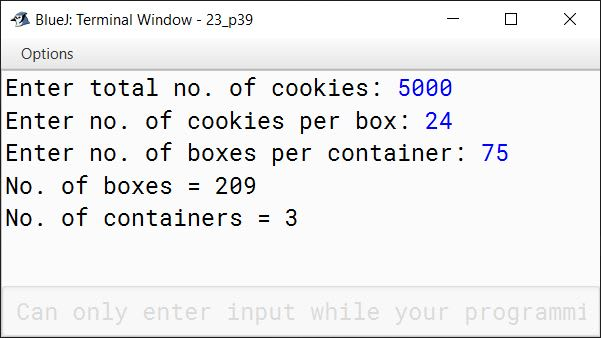#### Question 16

A special two-digit number is such that when the sum of its digits is added to the product of its digits, the result is equal to the original two-digit number.
Example: Consider the number 59.
Sum of digits = 5 + 9 = 14
Product of digits = 5 * 9 = 45
Sum of the sum of digits and product of digits = 14 + 45 = 59
Write a program to accept a two-digit number. Add the sum of its digits to the product of its digits. If the value is equal to the input number, display the message "Special 2 - digit number" otherwise, display the message "Not a special two-digit number".

import java.util.Scanner;

public class KboatSpecialNumber
{
public static void main(String args[]) {

Scanner in = new Scanner(System.in);

System.out.print("Enter a 2 digit number: ");
int orgNum = in.nextInt();

if (orgNum < 10 || orgNum > 99) {
System.out.println("Invalid input. Entered number is not a 2 digit number");
System.exit(0);
}

int num = orgNum;

int digit1 = num % 10;
int digit2 = num / 10;
num /= 10;

int digitSum = digit1 + digit2;
int digitProduct = digit1 * digit2;
int grandSum = digitSum + digitProduct;

if (grandSum == orgNum)
System.out.println("Special 2-digit number");
else
System.out.println("Not a special 2-digit number");

}
}
##### Output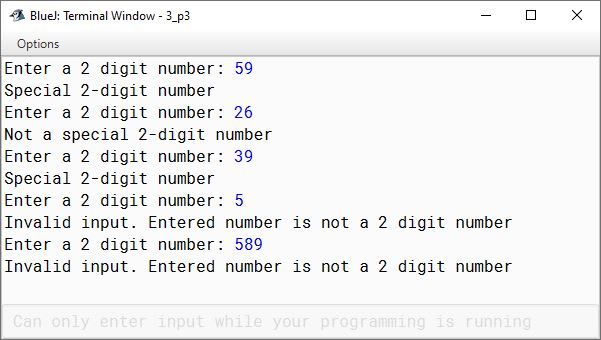#### Question 17

Using the switch statement, write a menu driven program:

1. To check and display whether a number input by the user is a composite number or not.
A number is said to be composite, if it has one or more than one factors excluding 1 and the number itself.
Example: 4, 6, 8, 9...
2. To find the smallest digit of an integer that is input:
Sample input: 6524
Sample output: Smallest digit is 2
For an incorrect choice, an appropriate error message should be displayed.

import java.util.Scanner;

public class KboatNumber
{
public static void main(String args[]) {
Scanner in = new Scanner(System.in);
System.out.println("Type 1 for Composite Number");
System.out.println("Type 2 for Smallest Digit");
int ch = in.nextInt();

switch (ch) {
case 1:
System.out.print("Enter Number: ");
int n = in.nextInt();
int c = 0;
for (int i = 1; i <= n; i++) {
if (n % i == 0)
c++;
}

if (c > 2)
System.out.println("Composite Number");
else
System.out.println("Not a Composite Number");
break;

case 2:
System.out.print("Enter Number: ");
int num = in.nextInt();
int s = 10;
while (num != 0) {
int d = num % 10;
if (d < s)
s = d;
num /= 10;
}
System.out.println("Smallest digit is " + s);
break;

default:
System.out.println("Wrong choice");
}
}
}
##### Output